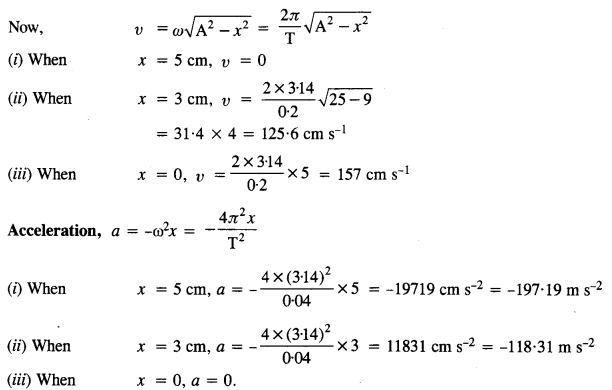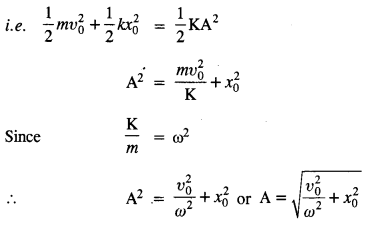## NCERT Solutions for Class 11 Physics Chapter 14 Oscillations

These Solutions are part of NCERT Solutions for Class 11 Physics. Here we have given NCERT Solutions for Class 11 Physics Chapter 14 Oscillations

Question 1.
Which of the following examples represent periodic motion?
(a) A swimmer completing one (return) trip from one bank of a river to the other and back.
(b) A freely suspended bar magnet displayed from its N-S direction and released.
(c) A hydrogen molecule rotating about its center of mass.
(d) An arrow released from a bow.
(b) and (c).
(Swimmer does not repeat trip after regular interval of time. Similarly, arrow released from a bow is not periodic).

Question 2.
Which of the following examples represent (nearly) simple harmonic motion and which represent periodic but not simple harmonic motion?
(a) the rotation of earth about its axis.
(b) motion of an oscillating mercury column in a U-tube.
(c) motion of a ball bearing inside a smooth curved bowl, when released from a point slightly above the lower most point.
(d) general vibrations of a polyatomic molecule about its equilibrium position.
(b) and (c) represent SHM in particular, (a) represents the period motion, (d) represents a superposition of a number of simple harmonic motions and is a periodic motion not necessarily S.H.M.

Question 3.
Figure depicts’four x-t plots for linear motion of a particle. Which of the plots represents periodic motion ? What is the period of motion (in case of periodic motion) ?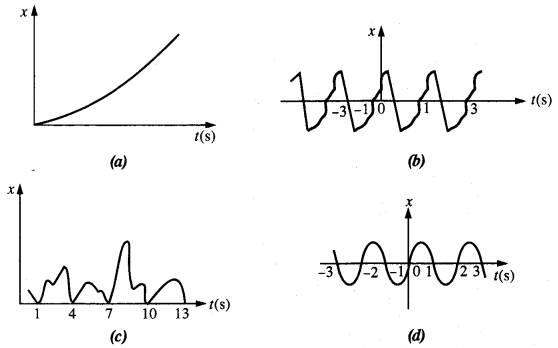Fig (b) and (d) represent periodic motions and the time period of each of these is 2 second, (a) and (c) are non periodic motions.

Question 4.
Which of the following functions of time represent (a) simple harmonic, (b) periodic but not simple harmonic, and (c) non-periodic motion ? Give period for each case of periodic motion : (ω is any positive constant).
(a) sin ωt – cos ωt
(b) sin2 ωt
(c) 3 cos (π/4 — 2ωt)
(d) cos ωt + cos 3ωt + cos 5ωt
(e) exp (- ω2t2 )
(f) 1 +ωt +ω2t2Question 5.
A particle is in linear simple harmonic motion between two points, A and B, 10 cm apart. Take the direction from A to B as the positive direction and give the signs of velocity, acceleration and force on the particle when it is (a) at the end A, (b) at the end B, (c) at the mid-point of AB going towards A, (d) at 2 cm away from B going towards A, (e) at 3 cm away from A going towards B, and (f) at 4 cm away from A going towards A.
A and B form the extreme positions and the center of line AB (let it be O) forms the mean position.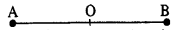(a) At the end A, e., extreme position, velocity is zero, acceleration and force are directed towards O and are positive.

(b) At the end B, e., second extreme position, velocity is zero whereas the acceleration and force are directed towards the point O and are negative.

(c) At the mid point 0, while going towards A, velocity is negative and maximum. The acceleration and force are both zero.

(d) At a distance 2 cm away from B going towards A, velocity is negative. Since acceleration and force are also directed towards O, both of them are negative.

(e) At a distance 3 cm away from A going towards B, velocity is positive. Since the acceleration and force are directed towards 0 from A, both of these are positive.

(f) At a distance of 4 cm away from A and going towards A, velocity is directed along BA, therefore, it is positive.Since acceleration and force are directed towards OB, both of them are positive.

Question 6.
Which of the following relationships between the acceleration a and the displacement x of a particle involve simple harmonic motion ?
(a) a = 0.7x           (b) a = – 200 x2        (c) a = -10x                   (d) a = 1003
Only the relation (c) represents the simple harmonic motion because for S.H.M., a a – x.

Question 7.
The motion of a particle executing simple harmonic motion is described by the displacement function, x(t) = A cos (cos ωt + Φ)
If the initial (t = 0) position of the particle is 1 cm and its initial velocity is co cm/s, what are its amplitude and initial phase angle ? The angular frequency of the particle is n s_1. If instead of the cosine function, we choose the sine function to describe the SHM : x = B sin (at + a), what are the amplitude and initial phase of the particle with the above initial conditions ?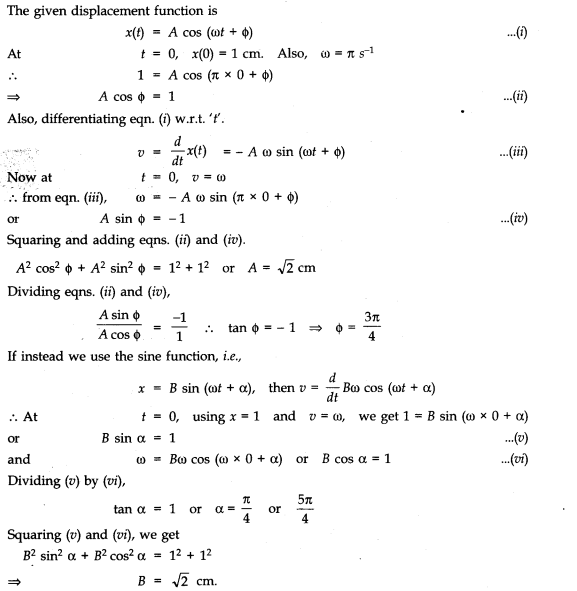Question 8.
A spring balance has a scale that reads from 0 to 50 kg. The length of the scale is 20 cm. A body suspended from this balance,,when displaced and released, oscillates with a period of 0.6 s. What is the weight of the body ?
Length of scale = 20 cm = 0.20 m
Total scale reading = 50 kg
∴ F = mg = 50 x 9.8 = 490 N
x= 20 cm = 0.20 m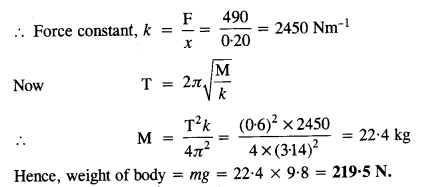Question 9.
A spring with a spring constant 1200 N m-1 is mounted on a horizontal table as shown in Fig. A mass of 3 kg is attached to the free end of the spring. The mass is then pulled sideways to a distance of 2.0 cm and released. Determine
(1) the frequency of oscillations,
(2) maximum acceleration of the mass, and
(3) the maximum speed of the mass.Here k = 1200 N m_1, m = 3 kg, A = 2.0 cm = 2 x 10-2 m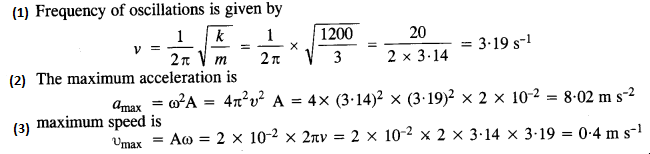Question 10.
In Exercise 9, let us take the position of mass when the spring is unstreched as x= 0, and the direction from left to right as the positive direction of x-axis. Give x as a function of time t for the oscillating mass if at the moment we start the stopwatch (t = 0), the mass is
(a) at the mean position,
(b) at the maximum stretched position, and
(c) at the maximum compressed position.
In what way do these functions for SHM differ from each other, in frequency, in amplitude or the initial phase ?
Here, the maximum displacement = Amplitude A = 2 cm
(a) If the time t = 0 at x = 0, the displacement can be expressed as the sine function of t.
x(t) = A sin ωt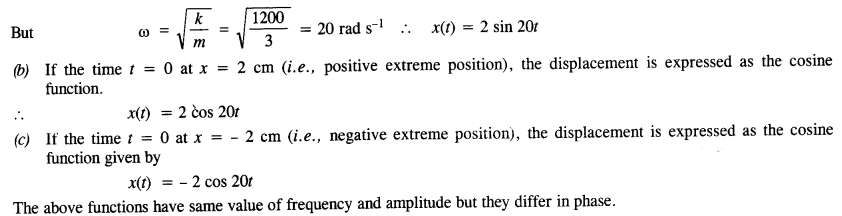Question 11.
Following figures correspond to two circular motions. The radius of the circle, the period of revolution, the initial position, and the sense of revolution (i.e clockwise or anti-clockwise) are indicated on each figure.Obtain the corresponding simple harmonic motions of the x-projection of the radius vector of the revolving particle P in each case.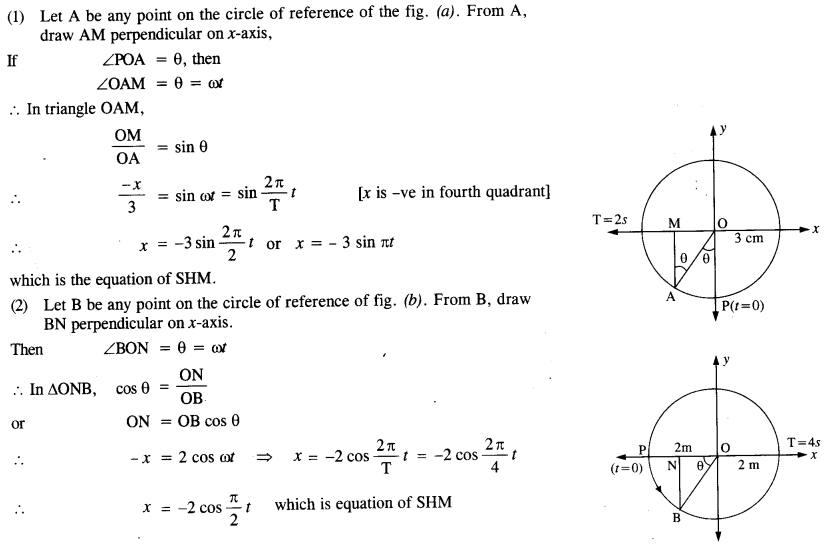Question 12.
Plot the corresponding reference circle for each of the following simple harmonic motions. Indicate the initial (t = 0) position of the particle, the radius of the circle, and the angular speed of the rotating particle. For simplicity, the sense of rotation may be fixed to be anticlockwise in every case (or is in cm and t is in s) :
(a) x = – 2 sin (3t + π/3)
(b) x = cos (π/6 – t)
(c) x = 3 sin (2nt + tc/4)
(d) x = 2 cos πt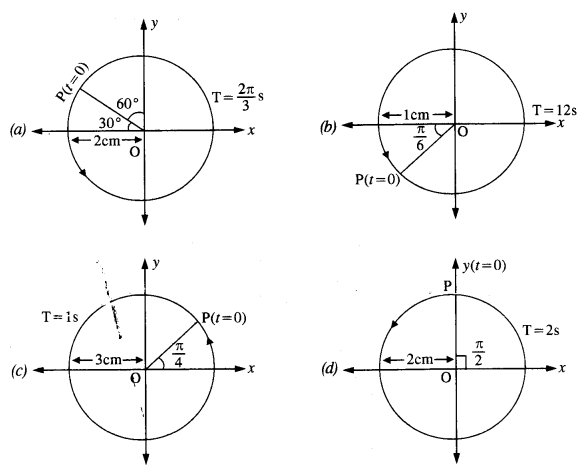Question 13.
Figure (a) shows a spring of force constant k clamped rigidly at one end and a mass m attached to its free end. A Figure (a) shows a spring of force constant k clamped rigidly at one end and a mass m attached to its free end. A force F applied at the free end stretches the spring. Figure (b) shows the same spring with both ends free and attached to a mass m at either end. Each end of the spring in Fig. (b) is stretched by the same force F.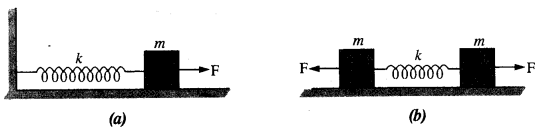(a) What is the maximum extension of the spring in the two cases ?
(b) If the mass in Fig. (a) and the two masses in Fig. (b) are released free, what is the period of oscillation in each case ?
(a) Let y be the maximum extension produced in the spring in fig. (a).
Then   F = Ay (in magnitude)   ∴y =  $$\frac { F }{ K }$$
In fig. (b), the force on one mass acts as the force of reaction due to the force on the other mass. Therefore, each mass behaves as if it is fixed with respect to the other.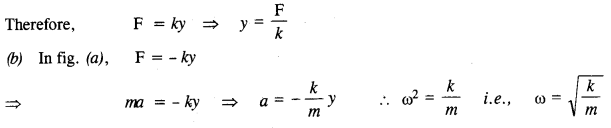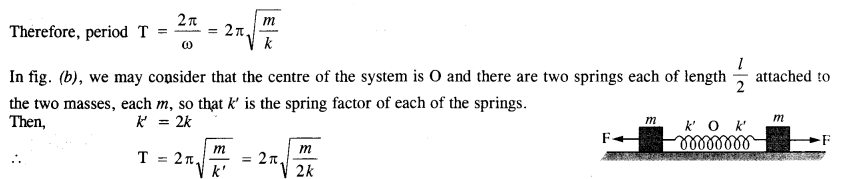Question 14.
The piston in the cylinder head of a locomotive has a stroke (twice the amplitude) of 1.0 m. If the piston moves with simple harmonic motion with an angular frequency of 200 rev/min,
what its maximum speed ?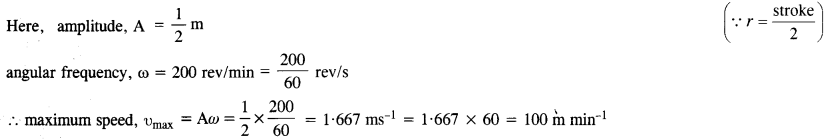Question 15.
The acceleration due to gravity on the surface of moon is 1.7 ms-2. What is the time period of a simple pendulum on the surface of moon if its time period on the surface of earth is 3.5 s ? (g on the surface of earth is 9.8 ms-2)Question 16.
Answer the following questions :
(a) Time period of a particle in SHM depends on the force constant k and mass m of the particle :A simple pendulum executes SHM approximately. Why then is the time period of a pendulum independent of the mass of the pendulum ?

(b) The motion of a simple pendulum is approximately simple harmonic for small angle oscillations. For larger angles of oscillation ,a more involved analysis shows that T is grater thanThink of a qualitative argument to appreciate this results.

(c) A man with a wristwatch on his hand falls from the top of a tower. Does the watch give correct time during the free fall ?

(d) What is the frequency of oscillation of a simple pendulum mounted in a cabin that is freely falling under gravity
In case of a spring, k does not depend upon m. However, in case of a simple pendulum, k is directly proportional to(c) The wrist watch uses an electronic system t>r spring system to give correct time, which does not change with acceleration due to gravity. Therefore, watch gives the correct time.

(d) During free fall of the cabin, the acceleration due to gravity is zero. Therefore, the frequency of oscillations is also zero e., the pendulum will not vibrate at all.

Question 17.

A simple pendulum of length l and having a bob of mass M is suspended in a car. The car is moving on a circular track of radius R with a uniform speed v. If the pendulum makes small oscillations in a radial direction about its equilibrium position, what will be its time period ?
In this case, the bob of the pendulum is under the action of two accelerations.
(1) Acceleration due to gravity ‘g’ acting vertically downwards.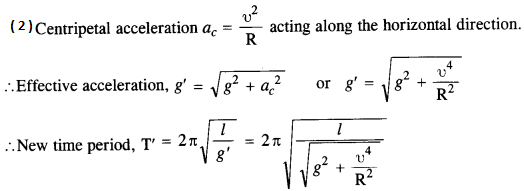Question 18.
A cylindrical piece of cork of base area A and height h floats in a liquid of density ρ. The cork is depressed slightly and then released. Show that the cork oscillates up and down simple harmonically with a period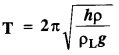where ρ is the density of cork. (Ignore damping due to viscosity of the liquid).
Let l be the height of the cork which is inside the liquid. Then, at equilibrium,
weight of the cork = upthrust of the portion of cork inside the liquid .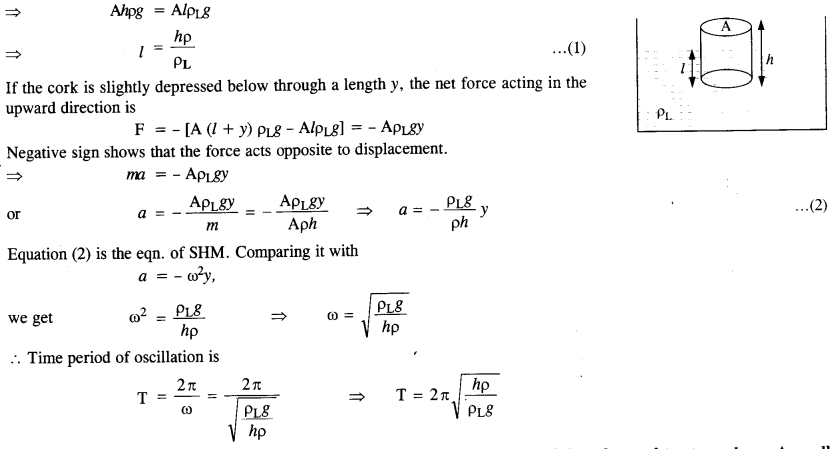Question 19.
One end of a U-tube containing mercury is connected to a suction pump and the other end to atmosphere. A small pressure difference is maintained between the two columns. Show that, when the suction pump is removed, the column of mercury in the U-tube executes simple harmonic motion.
The suction pump creates the pressure difference, thus mercury rises in one limb of the U-tube. When it is removed, a net force acts on the liquid column due to the difference in levels of mercury in the two limbs and hence the liquid column executes S.H.M. which can be expressed as:
Consider the mercury contained in a vertical U-tube upto the level P and Q in its two limbs.
Let P = density of the mercury.
L = Total length of the mercury column in both the limbs.
A = internal cross-sectional area of U-tube. m = mass of mercury in U-tube = LAP.
Assume, the mercury be depressed in left limb to F by a small distance y, then it rises by the same amount in the right limb to position Q’.
.’. Difference in levels in the two limbs = P’ Q’ = 2y.
:. Volume of mercury contained in the column of length 2y = A X 2y
.•. m – A x 2y x ρ.
If W = weight of liquid contained in the column of length 2y.
Then W = mg = A x 2y x ρ x g
This weight produces the restoring force (F) which tends to bring back the mercury to its equilibrium position.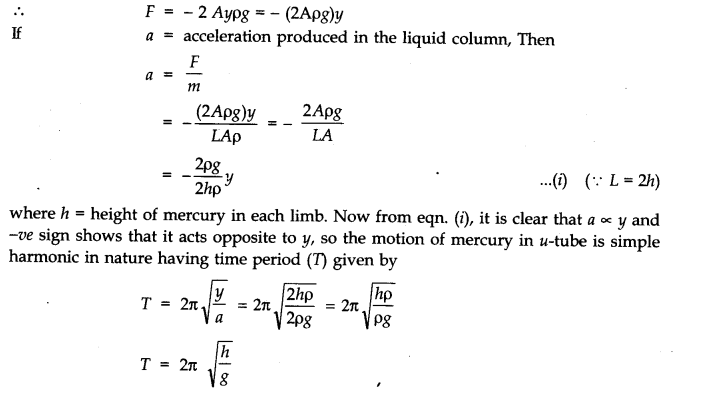Question 20.
An air chamber of volume V has a neck area of cross-section a into which a ball of mass m can move up and down without any friction (Fig.). Show that when the ball is pressed down a little and released, it executes SHM. Obtain an expression for the time period of oscillations assuming pressure-volume variations of air to be isothermal [see Fig.]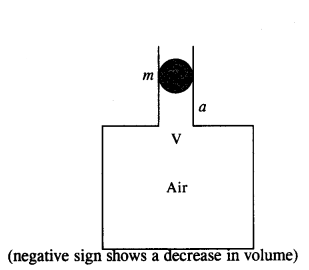When the ball is lying at rest in the long neck, the pressure of air below the ball inside the chamber is equal to the atmospheric pressure outside. If the ball is slightly depressed below through a distance y, then change in volume of air inside the chamber,Question 21.
You are riding in an automobile of mass 3000 kg. Assuming that you are examining the oscillation characteristics of its suspension system. The suspension sags 15 cm when the entire automobile is placed on it. Also, the amplitude of oscillation decreases by 50% during one complete oscillation. Estimate the values of (a) the spring constant k and (b) the damping constant b for the spring and shock absorber system of one wheel, assuming that each wheel supports 750 kg.
(a) Here, mass M = 3000 kg, displacement x = 15 cm = 015 m, g = 10 m/s2. There are four spring systems. If k is the spring constant of each spring, then total spring constant of all the four springs in parallel is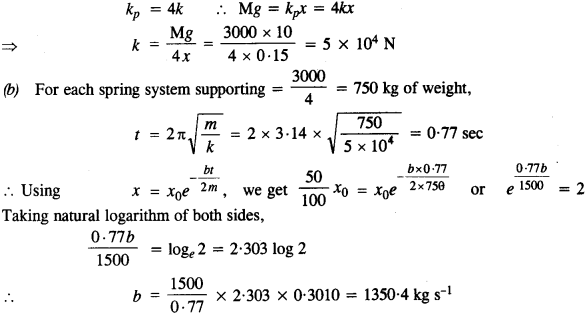Question 22.
Show that for a particle in linear SHM the average kinetic energy over a period of oscillation equals the average potential energy over the same period.
Let the SHM be represented as
y = r sin ωt, where r – amplitude…..(1)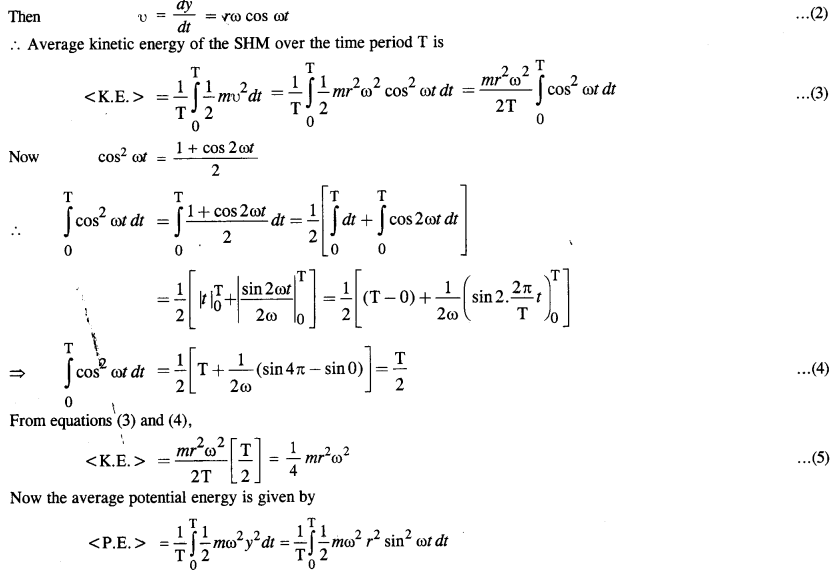Question 23.
A circular disc of mass 10 kg is suspended by a wire attached to its center. The wire is twisted by rotating the disc and released. The period of torsional oscillations is found to be 1.5 s. The radius of the disc is 15 cm. Determine the torsional spring constant of the wire. (Torsional spring constant a is defined by the relation J = – αθ, where J is the restoring couple and 0 the angle of twist).
Here, mass of disc, m = 10 kg,
radius r =15 cm = 0.15 m Tortional spring constant a = ?
Time period of tortional oscillations, T = 1.5 s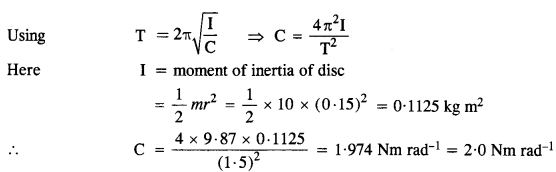Question 24.
A body describes a S.H.M. with an amplitude of 5 cm and period of 0.2 s. Find the acceleration and velocity of the body, when displacement is (a) 5 cm, (b) 3 cm, (c) 0 cm.